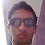## Monday, June 04, 2007

### MCQ from Electrostatics- Charged Spherical ShellsTwo thin concentric spherical shells of copper having radii R1 and R2 (R2 > R1) carry charges Q1 and Q2 respectively. The potential at a point P (Fig) distant ‘r’ from the common centre and located in between the shells is

(a) (1/4πε0)(Q1/r)

(b) (1/4πε0)(Q1 + Q2)/r

(c) (1/4πε0)[(Q1/R1)+ (Q2/R2)]

(d) (1/4πε0)[(Q1/R1) + (Q2/r)]

(e) (1/4πε0)[(Q1/r) + (Q2/R2)]

Potentials due to the two charged shells get added to give the net potential at P. The potential at P due to the smaller shell of radius R1 is (1/4πε0)(Q1/r) since the point P is outside the shell at distance ‘r’ from its centre. The potential at P due to the larger shell of radius R2 is (1/4πε0)(Q2/R2) since the point P is inside this shell and the potential everywhere inside is the same as the potential at the surface of the shell. The net potential at P is obtained by adding these two potentials.

Therefore, the net potential at P = (1/4πε0)[(Q1/r) + (Q2/R2)]

[This question can be modified by making one of the charges or both charges negative. You will then have to substitute the charges with the proper sign in the above equation].

What is the electric potential at a point inside the smaller shell?

The point is then inside both shells and both produce constant potentials (1/4πε0)(Q1/R1) and (1/4πε0)(Q2/R2) respectively. The net potential is therefore (1/4πε0)[(Q1/R1)+ (Q2/R2)]

What is the electric potential at a point outside the larger shell?

The point is then outside both shells and they produce potentials (1/4πε0)[(Q1/r) and (1/4πε0)[(Q2/r) respectively so that the net potential is (1/4πε0)(Q1 + Q2)/r. This is a very simple case since you can treat the entire charge Q1 + Q2 to be located at the common centre of the shells.

Now, one more question: What is the electric field at a point P in between the two shells?

The larger shell does not produce any field at P since it is inside and there is no potential gradient inside. The field at P is therefore due to the smaller shell only and is equal to (1/4πε0)[(Q1/r2)

You can find more posts on electrostatics by clicking on the label 'electrostatics' below this post or by performing a search for 'electrostatics' making use of the blog search box on this page.

1.Thanks for the post on Tech4Teaching. Your site is very cool. The diagrams are very nice. I studied engineering in college so I appreciate the challenging sciences.

Laura B. Fogle

2.Thank you very much for your words of appreciation.

3.I find your blog very useful in my preparation for IITJEE. I have a few doubts which i would like you to clarify on various topics. Could you kindly help me in giving me proper guidance. Since I do not have your email address i'm not able to reach you. I'll be too happy if you can respond to my address: sundar.vinod@gmail.com

SHYAM SUNDAR,
Chennai

4.Thank you Shyam.
You may use this comment option to express your doubts so that other visitors also will be benefitted.

5.sir,
its really nice.
i want to ask a question.
Q: there are four shells A, B, C and Dof radii a, 2a, 3a,and 4a respectively. shell B AND D are given charges+q and -q respectively. shell C is now earthened. the potetial difference VA-VB IS ???

6.Hello ric,
I presume that the shells are concentric. The shells A and B are inside the shells C and D. Since A is uncharged, the potentials of A and B are equal so that the potential difference between A and B is zero.

7.sir its too good

8.Thanks for the info, I didn't know that the larger shell does not produce any field at P. Nice and useful article, keep going!